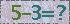2019年JOS入选“中国科技期刊卓越行动计划”
J. Semicond. > Volume 37 > Issue 3 > Article Number: 035002

# A SHA-less 14-bit, 100-MS/s pipelined ADC with comparator offset cancellation in background

Xiaofei Wang 1, , , Hong Zhang 2, , Jie Zhang 2, , Xin Du 2, and Yue Hao 1,

Abstract: A 14-bit 100-MS/s pipelined analog-to-digital converter(ADC) without dedicated front-end sample-and-hold amplifier(SHA) is presented. In addition to elaborate matching of the sampling network in the first stage, a background offset cancellation circuit is proposed in this paper to suppress the offset of the comparators in the first-stage sub-ADC, which ensures the overall offset does not exceed the correction range of the built-in redundant structure. Fabricated in a 0.18-μm CMOS technology, the presented ADC occupies a chip area of 12 mm2, and consumes 237 mW from a 1.8-V power supply. Measurement results with a 30.1-MHz input sine wave under a sampling rate of 100 MS/s show that the ADC achieves a 71-dB signal-to-noise and distortion ratio(SNDR), an 85.4-dB spurious-free dynamic range(SFDR), a maximum differential nonlinearity(DNL) of 0.22 LSB and a maximum integral nonlinearity(INL) of 1.4 LSB.

Abstract: A 14-bit 100-MS/s pipelined analog-to-digital converter(ADC) without dedicated front-end sample-and-hold amplifier(SHA) is presented. In addition to elaborate matching of the sampling network in the first stage, a background offset cancellation circuit is proposed in this paper to suppress the offset of the comparators in the first-stage sub-ADC, which ensures the overall offset does not exceed the correction range of the built-in redundant structure. Fabricated in a 0.18-μm CMOS technology, the presented ADC occupies a chip area of 12 mm2, and consumes 237 mW from a 1.8-V power supply. Measurement results with a 30.1-MHz input sine wave under a sampling rate of 100 MS/s show that the ADC achieves a 71-dB signal-to-noise and distortion ratio(SNDR), an 85.4-dB spurious-free dynamic range(SFDR), a maximum differential nonlinearity(DNL) of 0.22 LSB and a maximum integral nonlinearity(INL) of 1.4 LSB.

References:

  Chen Y, Chen C, Feng Z. 14-bit 100 MS/s 121 mW pipelined ADC[J]. Journal of Semiconductors, 2015, 36(6): 065008.  Zhao N, Luo H, Wei Q. A 14-bit 100-MS/s 85[J]. Journal of Semiconductors, 2014, 35(7).  Mehr I, Singer L. A 55-mW, 10-bit, 40-Msample/s Nyquist-rate CMOS ADC[J]. IEEE J Solid-State Circuits, 2000, 35(3): 318.  Devarajan S, Singer L, Kelly D. A 16-bit, 125 MS/s, 385 mW, 78[J]. IEEE J Solid-State Circuits, 2009, 44(12): 3305.  Huang P, Hsien S, Lu V. SHA-less pipelined ADC with in situ background clock-skew calibration[J]. IEEE J Solid-State Circuits, 2011, 46(8): 1893.  Ali A, Dillon C, Sneed R. A 14-bit 125 MS/s IF/RF sampling pipelined ADC with 100 dB SFDR and 50 fs jitter[J]. IEEE J Solid-State Circuits, 2006, 41(8): 1846.  Ali A, Dinc H, Bhoraskar P. A 14 bit 1 GS/s RF sampling pipelined ADC with background calibration[J]. IEEE J Solid-State Circuits, 2014, 49(12): 2857.  Plas G, Decoutere S, Donnay S. A 0[J]. IEEE International Solid-State Circuits Conference Digest of Technical Papers(ISSCC), 2006: 566.  Miyahara M, Asada Y, Paik D. A low-noise self-calibrating dynamic comparator for high-speed ADCs[J]. Proc IEEE Asia Solid-State Circuits Conference(A-SSCC), 2008: 269.  Wang K, Fan C, Zhou J. A 14-bit 100 MS/s CMOS pipelined ADC with 11[J]. Journal of Semiconductors, 2013, 34(8).
  Chen Y, Chen C, Feng Z. 14-bit 100 MS/s 121 mW pipelined ADC[J]. Journal of Semiconductors, 2015, 36(6): 065008.  Zhao N, Luo H, Wei Q. A 14-bit 100-MS/s 85[J]. Journal of Semiconductors, 2014, 35(7).  Mehr I, Singer L. A 55-mW, 10-bit, 40-Msample/s Nyquist-rate CMOS ADC[J]. IEEE J Solid-State Circuits, 2000, 35(3): 318.  Devarajan S, Singer L, Kelly D. A 16-bit, 125 MS/s, 385 mW, 78[J]. IEEE J Solid-State Circuits, 2009, 44(12): 3305.  Huang P, Hsien S, Lu V. SHA-less pipelined ADC with in situ background clock-skew calibration[J]. IEEE J Solid-State Circuits, 2011, 46(8): 1893.  Ali A, Dillon C, Sneed R. A 14-bit 125 MS/s IF/RF sampling pipelined ADC with 100 dB SFDR and 50 fs jitter[J]. IEEE J Solid-State Circuits, 2006, 41(8): 1846.  Ali A, Dinc H, Bhoraskar P. A 14 bit 1 GS/s RF sampling pipelined ADC with background calibration[J]. IEEE J Solid-State Circuits, 2014, 49(12): 2857.  Plas G, Decoutere S, Donnay S. A 0[J]. IEEE International Solid-State Circuits Conference Digest of Technical Papers(ISSCC), 2006: 566.  Miyahara M, Asada Y, Paik D. A low-noise self-calibrating dynamic comparator for high-speed ADCs[J]. Proc IEEE Asia Solid-State Circuits Conference(A-SSCC), 2008: 269.  Wang K, Fan C, Zhou J. A 14-bit 100 MS/s CMOS pipelined ADC with 11[J]. Journal of Semiconductors, 2013, 34(8).

## GET CITATION

X F Wang, H Zhang, J Zhang, X Du, Y Hao. A SHA-less 14-bit, 100-MS/s pipelined ADC with comparator offset cancellation in background[J]. J. Semicond., 2016, 37(3): 035002. doi: 10.1088/1674-4926/37/3/035002.

Export: BibTex EndNote

## Article Metrics

Article views: 1689 Times PDF downloads: 40 Times Cited by: 0 Times

## History

Manuscript received: 25 July 2015 Manuscript revised: Online: Published: 01 March 2016

Code:*验证码错误